NCERT Solutions: Lines & Angles

# NCERT Solutions: Lines & Angles Notes | Study Mathematics (Maths) Class 7 - Class 7

## Document Description: NCERT Solutions: Lines & Angles for Class 7 2022 is part of Mathematics for Mathematics (Maths) Class 7 preparation. The notes and questions for NCERT Solutions: Lines & Angles have been prepared according to the Class 7 exam syllabus. Information about NCERT Solutions: Lines & Angles covers topics like Exercise 5.1, Exercise 5.2&nbsp; and NCERT Solutions: Lines & Angles Example, for Class 7 2022 Exam. Find important definitions, questions, notes, meanings, examples, exercises and tests below for NCERT Solutions: Lines & Angles.

Introduction of NCERT Solutions: Lines & Angles in English is available as part of our Mathematics (Maths) Class 7 for Class 7 & NCERT Solutions: Lines & Angles in Hindi for Mathematics (Maths) Class 7 course. Download more important topics related with Mathematics, notes, lectures and mock test series for Class 7 Exam by signing up for free. Class 7: NCERT Solutions: Lines & Angles Notes | Study Mathematics (Maths) Class 7 - Class 7
 1 Crore+ students have signed up on EduRev. Have you?

Exercise 5.1

Q1. Find the complement of each of the following angles:Ans: Two angles are said to be complementary if the sum of their measures is 90º.
The given angle is 20º
Let the measure of its complement be xº.
Then,
= x + 20º = 90º
= x = 90º – 20º
= x = 70º
Hence, the complement of the given angle measures 70º.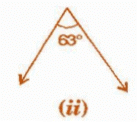Ans: Two angles are said to be complementary if the sum of their measures is 90º.
The given angle is 63º
Let the measure of its complement be xº.
Then,
= x + 63º = 90º
= x = 90º – 63º
= x = 27º
Hence, the complement of the given angle measures 27º.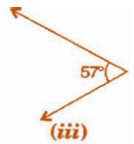Ans: Two angles are said to be complementary if the sum of their measures is 90º.
The given angle is 57º
Let the measure of its complement be º.
Then,
= x + 57º = 90º
= x = 90º – 57º
= x = 33º
Hence, the complement of the given angle measures 33º.

Q2. Find the supplement of each of the following angles: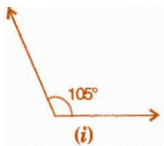Ans: Two angles are said to be supplementary if the sum of their measures is 180º.
The given angle is 105º
Let the measure of its supplement be xº.
Then,
= x + 105º = 180º
= x = 180º – 105º
= x = 75º
Hence, the supplement of the given angle measures 75º.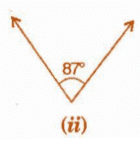Ans: Two angles are said to be supplementary if the sum of their measures is 180º.
The given angle is 87º
Let the measure of its supplement be xº.
Then, = x + 87º = 180º
= x = 180º – 87º
= x = 93º
Hence, the supplement of the given angle measures 93º.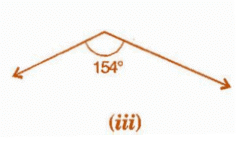Ans: Two angles are said to be supplementary if the sum of their measures is 180º.
The given angle is 154º
Let the measure of its supplement be xº.
Then,
= x + 154º = 180º
= x = 180º – 154º
= x = 26º
Hence, the supplement of the given angle measures 93º.

Q3. Identify which of the following pairs of angles are complementary and which are supplementary:

(i) 65°, 115°
Ans: We have to find the sum of given angles to identify whether the angles are complementary or supplementary.
Then,
= 65º + 115º
= 180º
If the sum of two angle measures is 180º, then the two angles are said to be supplementary.
∴ These angles are supplementary angles.

(ii) 63°, 27°
Ans: We have to find the sum of given angles to identify whether the angles are complementary or supplementary.
Then,
= 63º + 27º
= 90º
If the sum of two angle measures is 90º, then the two angles are said to be complementary.
∴ These angles are complementary angles.

(iii) 112°, 68°
Ans: We have to find the sum of given angles to identify whether the angles are complementary or supplementary.
Then,
= 112º + 68º
= 180º
If the sum of two angle measures is 180º, then the two angles are said to be supplementary.
∴ These angles are supplementary angles.

(iv) 130°, 50°
Ans: We have to find the sum of given angles to identify whether the angles are complementary or supplementary.
Then,
= 130º + 50º
= 180º
If the sum of two angle measures is 180º, then the two angles are said to be supplementary.
∴ These angles are supplementary angles.

(v)  45°, 45°
Ans: We have to find the sum of given angles to identify whether the angles are complementary or supplementary.
Then, = 45º + 45º = 90º
If the sum of two angle measures is 90º, then the two angles are said to be complementary.
∴ These angles are complementary angles.

(vi) 80°,10°
Ans: We have to find the sum of given angles to identify whether the angles are complementary or supplementary.
Then, = 80º + 10º = 90º
If the sum of two angle measures is 90º, then the two angles are said to be complementary.
∴ These angles are complementary angles.

Q4. Find the angle which is equal to its complement.
Ans: Let one of the two equal complementary angles be x.
∴ x + x = 90°
⇒ 2x = 90°
⇒ x = 90°/2 = 45°
Thus, 45° is equal to its complement.

Q5. Find the angle which is equal to its supplement.
Ans: Let x be two equal angles of its supplement.
Therefore,
x + x = 180° [Supplementary angles]
⇒ 2x = 180°
⇒ x = 180°/2 = 90°
Thus, 90° is equal to its supplement.

Q6. In the given figure,1 and 2 are supplementary angles. If 1 is decreased, what changes should take place in 2 so that both the angles still remain supplementary?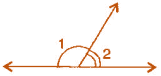Ans: If ∠1 is decreased then, ∠2 will increase with the same measure, so that both the angles still remain supplementary.

Q7. Can two angles be supplementary if both of them are:

(i) acute
Ans: No. If two angles are acute, means less than 90°, the two angles cannot be supplementary. Because, their sum will be always less than 90°.

(ii) obtuse
Ans: No. If two angles are obtuse, means more than 90°, the two angles cannot be supplementary. Because, their sum will be always more than 180°.

(iii) right?
Ans: Yes. If two angles are right, means both measures 90°, then two angles can form a supplementary pair.
∴ 90° + 90° = 180

Q8. An angle is greater than 45o . Is its complementary angle greater than 45o or equal to 45o or less than 45o ?
Ans: Let the complementary angles be x and y, i.e.p x+ y = 90°
It is given that x > 45°
Adding y both sides,  x + y > 45° + y
⇒ 90° > 45° + y
⇒ 90 - 45° > y
⇒ y < 45°
Thus, its complementary angle is less than 45°.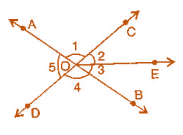(i) Is ∠ 1 adjacent to ∠21
Ans: By observing the figure we came to conclude that,
Yes, as ∠1 and ∠2 having a common vertex i.e. O and a common arm OC.
Their non-common arms OA and OE are on both the side of common arm.

(ii) Is ∠AOC adjacent to ∠AOE?
Ans: By observing the figure, we came to conclude that,
No, since they are having a common vertex O and common arm OA.
But, they have no non-common arms on both the side of the common arm.

(iii) Do ∠COE and ∠EOD form a linear pair?
Ans: By observing the figure, we came to conclude that,
Yes, as ∠COE and ∠EOD having a common vertex i.e. O and a common arm OE.
Their non-common arms OC and OD are on both the side of common arm.

(iv) Are ∠BOD and ∠DOA supplementary?
Ans: By observing the figure, we came to conclude that,
Yes, as ∠BOD and ∠DOA having a common vertex i.e. O and a common arm OE.
Their non-common arms OA and OB are opposite to each other.

(v)  Is ∠1 vertically opposite to ∠4?
Ans: Yes, ∠1 and ∠2 are formed by the intersection of two straight lines AB and CD.

(vi) What is the vertically opposite angle of ∠5?
Ans: ∠COB is the vertically opposite angle of ∠5. Because these two angles are formed by the intersection of two straight lines AB and CD.

Q10. Indicate which pairs of angles are: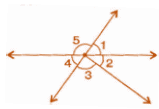(i) Vertically opposite angles?
Ans: By observing the figure we can say that,
∠1 and ∠4, ∠5 and ∠2 + ∠3 are vertically opposite angles. Because these two angles are formed by the intersection of two straight lines.

(ii) Linear pairs?
Ans: By observing the figure we can say that,
∠1 and ∠5, ∠5 and ∠4 as these are having a common vertex and also having non common arms opposite to each other.

Q11. In the following figure, is ∠1 adjacent to ∠2? Give reasons.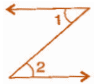Ans: 1 and 2 are not adjacent angles because their vertex is not common.

Q12. Find the values of the angles x,y and z in each of the following: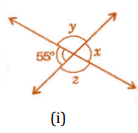Ans: x = 55°  [Vertically opposite angles]
Now 55°+ y = 180°   [Linear pair]
⇒ y = 180° - 55° = 125°
Also y = z = 125° [Vertically opposite angles]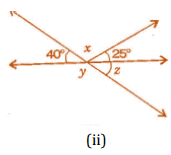Ans: 40°+ x + 25° = 180°  [Angles on straight line]
⇒ 65°+ x = 180°
⇒ x = 180°- 65° = 115°
Now 40° + y = 180° [Linear pair]
⇒ y -180° -  40° = 140° ........(i)
Also y + z = 180°  [Linear pair]
⇒ 140°+r = 180°  [From equation (i)]
⇒ z = 180°-140° = 40°
Thus, x = 115°,y = 140° and z = 40°.

Q13. Fill in the blanks:

(i) If two angles are complementary, then the sum of their measures is _______________.
Ans: If two angles are complementary, then the sum of their measures is 90°.

(ii) If two angles are supplementary, then the sum of their measures is _______________.
Ans: If two angles are supplementary, then the sum of their measures is 180°.

(iii) Two angles forming a linear pair are _______________.
Ans: Two angles forming a linear pair are Supplementary.

(iv) If two adjacent angles are supplementary, they form a _______________.
Ans: If two adjacent angles are supplementary, they form a linear pair.

(v) If two lines intersect a point, then the vertically opposite angles are always _______________.
Ans: If two lines intersect at a point, then the vertically opposite angles are always equal.

(vi) If two lines intersect at a point and if one pair of vertically opposite angles are acute angles, then the other pair of vertically opposite angles are _______________.
Ans: If two lines intersect at a point, and if one pair of vertically opposite angles are acute angles, then the other pair of vertically opposite angles are Obtuse angles.

Q14. In the adjoining figure, name the following pairs of angles: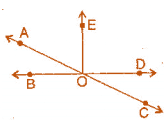(i) Obtuse vertically opposite angles.
Ans: Obtuse vertically opposite angles means greater than 90’ and equal ∠AOD = ∠BOC.

Ans: Adjacent complementary angles means angles have common vertex, common arm, non-common arms are on either side of common arm and sum of angles is 90°.

(iii) Equal supplementary angles.
Ans: Equal supplementary angles means sum of angles is 180° and supplement angles are equal.

(iv) Unequal supplementary angles.
Ans: Unequal supplementary angles means sum of angles is 180° and supplement angles are unequal.
i.e.,  ∠AOE, ∠EOC;  ∠AOD, ∠DOC and ∠AOB, ∠BOC

(v) Adjacent angles that do not form a linear pair.
Ans: Adjacent angles that do not form a linear pair mean, angles have common ray but the angles in a linear pair are not supplementary.
i.e.,  ∠AOB, ∠AOE;  ∠AOE, ∠EOD and ∠EOD, ∠COD

Exercise 5.2

Q1. State the property that is used in each of the following statements: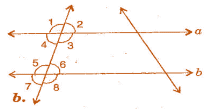(i) If a║b, then ∠1 = ∠5.
Ans: Given,   a║b, then ∠1 = ∠5    [Corresponding angles]
If two parallel lines are cut by a transversal, each pair of corresponding angles are equal in measure.

(ii) If ∠4 = ∠6, then a║b.
Ans: Given, ∠4 = ∠6, then a║b   [Alternate interior angles]
When a transversal cuts two lines such that pairs of alternate interior angles are equal, the lines have to be parallel.

(iii) If ∠4 + ∠5 + 180°, then a║b
Ans: Given,  ∠4+ ∠5= 180°, then a║b   [Co-interior Angles]
When a transversal cuts two lines, such that pairs of interior angles on the same side of transversal are supplementary, the lines have to be parallel.

Q2. In the adjoining figure, identify: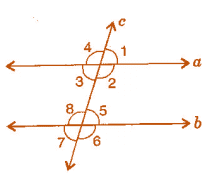(i) the pairs of corresponding angles.
Ans: The pairs of corresponding angles: ∠1, ∠5; ∠2, ∠6; ∠4, ∠8 and ∠3, ∠7

(ii) the pairs of alternate interior angles.
Ans: The pairs of alternate interior angles are: ∠3, ∠5 and ∠2, ∠8

(iii) the pairs of interior angles on the same side of the transversal.
Ans: The pair of interior angles on the same side of the transversal: ∠3, ∠8 and ∠2, ∠5

(iv) the vertically opposite angles.
Ans: The vertically opposite angles are: ∠1, ∠3; ∠2, ∠4; ∠6, ∠8 and ∠5, ∠7

Q3. In the adjoining figure, p║q. Find the unknown angles.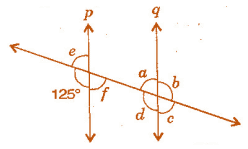Ans: Given, p║q and cut by a transversal line,
∵ 125°+ e = 180°    [Linear pair]
∵ e = 180°-125° = 55°   ....(i)
Now  e = f = 55°   [Vertically opposite angles]
Also  a = f = 55°   [Alternate interior angles]
a + b = 180°    [Linear pair]
⇒  55° + b = 180°    [From equation (i)]
⇒  6 = 180°- 55°=    125°
Now  a = c = 55° and    b=d = 125°    [Vertically opposite angles]
Thus, a = 55°,b = 125°,c = 55°, d = 125°, e = 55° and f = 55°.

Q4. Find the values of x in each of the following figures if l || m.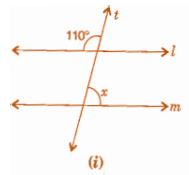Ans: Let us assume other angle on the line m be ∠y,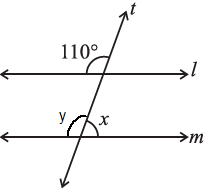Then, By the property of corresponding angles,∠y = 110°
We know that Linear pair is the sum of adjacent angles is 180°
Then,
= ∠x + ∠y = 180°
= ∠x + 110° = 180°
= ∠x = 180° – 110°
= ∠x = 70°
(ii)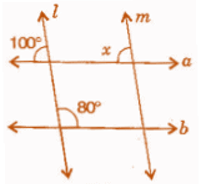Ans: By the property of corresponding angles,
∠x = 100°

Q5. In the given figure, the arms of two angles are parallel. If ΔABC = 70o , then find: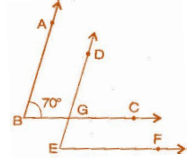(i) DGC
Ans: Given, AB ║ DE and BC is a transversal line and ∠ABC = 70°
∴  ∠ABC = ∠DGC    [Corresponding angles]
∠ DGC = 70°  ........(i)

(ii) DEF
Ans: Given, BC ║ EF and DE is a transversal line and ∠DGC = 70°
∴ ∠DGC = ∠DEF    [Corresponding angles]
∠ DEF = 70°    [From equation (i)]

Q6. In the given figures below, decide whether l is parallel to m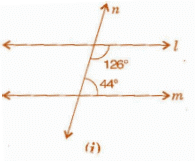Ans: Let us consider the two lines l and m, n is the transversal line intersecting l and m.
We know that the sum of interior angles on the same side of transversal is 180°.
Then,
= 126° + 44°
= 170°
But, the sum of interior angles on the same side of transversal is not equal to 180°.
So, line l is not parallel to line m.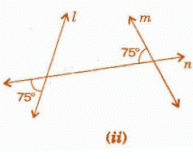Ans: Let us assume ∠x be the vertically opposite angle formed due to the intersection of the straight line l and transversal n,
Then, ∠x = 75°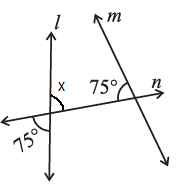Let us consider the two lines l and m,
n is the transversal line intersecting l and m.
We know that the sum of interior angles on the same side of transversal is 180°.
Then,
= 75° + 75°
= 150°
But, the sum of interior angles on the same side of transversal is not equal to 180o.
So, line l is not parallel to line m.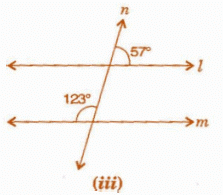Ans: Let us assume ∠x be the vertically opposite angle formed due to the intersection of the Straight line l and transversal line n,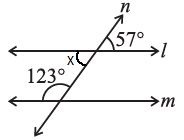Let us consider the two lines l and m, n is the transversal line intersecting l and m.
We know that the sum of interior angles on the same side of transversal is 180°.
Then,
= 123° + ∠x
= 123° + 57°
= 180°
∴ The sum of interior angles on the same side of transversal is equal to 180°.
So, line l is parallel to line m.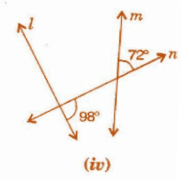Ans: Let us assume ∠x be the angle formed due to the intersection of the Straight line l and transversal line n,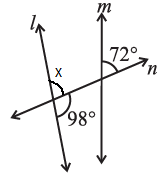We know that Linear pair is the sum of adjacent angles is equal to 180°.
= ∠x + 98° = 180°
= ∠x = 180° – 98°
= ∠x = 82°
Now, we consider ∠x and 72° are the corresponding angles.
For l and m to be parallel to each other, corresponding angles should be equal.
But, in the given figure corresponding angles measures 82° and 72° respectively.
∴ Line l is not parallel to line m.

The document NCERT Solutions: Lines & Angles Notes | Study Mathematics (Maths) Class 7 - Class 7 is a part of the Class 7 Course Mathematics (Maths) Class 7.
All you need of Class 7 at this link: Class 7

## Mathematics (Maths) Class 7

166 videos|252 docs|45 tests
 Use Code STAYHOME200 and get INR 200 additional OFF

## Mathematics (Maths) Class 7

166 videos|252 docs|45 tests

Track your progress, build streaks, highlight & save important lessons and more!

,

,

,

,

,

,

,

,

,

,

,

,

,

,

,

,

,

,

,

,

,

;